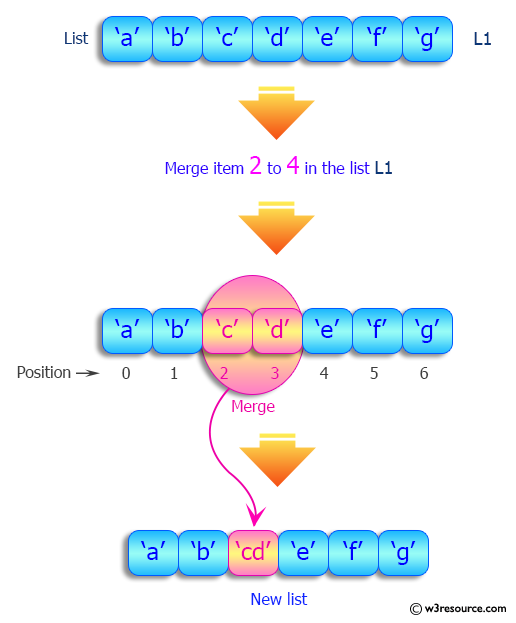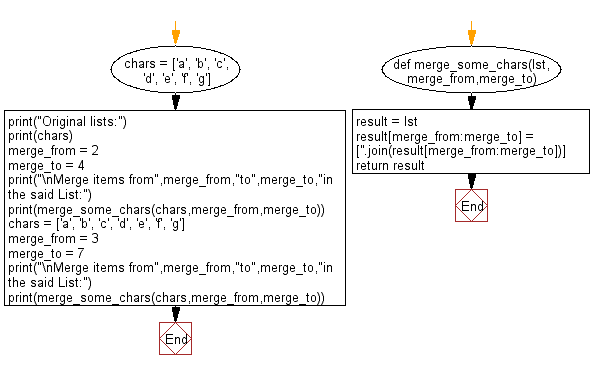﻿ Python: Merge some list items in given list using index value - w3resource# Python: Merge some list items in given list using index value

## Python List: Exercise - 173 with Solution

Write a Python program to merge some list items in given list using index value.

Sample Solution:

Python Code:

``````def merge_some_chars(lst,merge_from,merge_to):
result = lst
result[merge_from:merge_to] = [''.join(result[merge_from:merge_to])]
return result
chars = ['a', 'b', 'c', 'd', 'e', 'f', 'g']
print("Original lists:")
print(chars)
merge_from = 2
merge_to = 4
print("\nMerge items from",merge_from,"to",merge_to,"in the said List:")
print(merge_some_chars(chars,merge_from,merge_to))
chars = ['a', 'b', 'c', 'd', 'e', 'f', 'g']
merge_from = 3
merge_to = 7
print("\nMerge items from",merge_from,"to",merge_to,"in the said List:")
print(merge_some_chars(chars,merge_from,merge_to))
```
```

Sample Output:

```Original lists:
['a', 'b', 'c', 'd', 'e', 'f', 'g']

Merge items from 2 to 4 in the said List:
['a', 'b', 'cd', 'e', 'f', 'g']

Merge items from 3 to 7 in the said List:
['a', 'b', 'c', 'defg']
```

Pictorial Presentation:Flowchart:## Visualize Python code execution:

The following tool visualize what the computer is doing step-by-step as it executes the said program:

Python Code Editor:

Have another way to solve this solution? Contribute your code (and comments) through Disqus.

What is the difficulty level of this exercise?

Test your Python skills with w3resource's quiz

﻿

## Python: Tips of the Day

```print(2_000_000)
```2000000